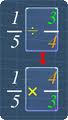• Use the sites below to help you practice each of the skills listed.

DivisionSnork's Long Division Game
A step by step long division game that allows students to practice long division skills.  Students get to teach a lovable monster named Snork how to divide.

Draggable Division
Drag large or small numbers onto graph paper to show the steps to long division.  You choose ho many digits in the divisor and dividend.

Prime Factorization
Watch this video to review how to find the prime factorization of a number.

Greatest Common Factor
This video shows how to find the GCF using listing factors and the Cake Method.  The video calls the Cake Method the Ladder Method, but it is the same process we used in class.

Least Common Multiple
This video shows how to use the listing multiples method for finding the LCM as well as the Cake Method.  The video calls the Cake Method the Ladder Method, but it is the same technique we used in class.

Greatest Common Factor Word Problems
This video helps to show you when to use GCF when solving a word problem.

Multiplying/Dividing FractionsMultiplying Fractions Practice
In order to become skilled in math, you need to practice, practice, practice!

Multiplying Fractions -   Watch to see a sample of multiplying two fractions with and without cross canceling.

Brownie Pan Powerpoint: This is the powerpoint shown in class that models how to use the Brownie Pan method to multiply fractions.

Multiplying Mixed Numbers -  Sample problem for multiplying a mixed number by a mixed number.

Dividing Fractions - Sample problem for dividing a fraction by a fraction.  Keep, Switch, Flip

Dividing Fractions Practice
Attempt to solve 8 correct and then move on to practice more.  If not, review and try again.

Dividing Mixed Numbers - Sample problem for dividing a mixed number by a mixed number.

Soccer Shootout
Practice your skills in adding, subtracting, multiplying, and dividing fractions.  Choose from 4 different levels.

DecimalsInteractive Decimal Games

Multiplying and Dividing Decimals

Adding, Subracting, Multiplying, and Dividing Decimals

Adding and Subtracting Positive and Negative IntegersReview the rules for adding and subtracting integers and then try playing "Casey Runner" to practice your skills with integers!

Walk the Plank
Answer questions on adding and subtracting integers.  Get it wrong, and you take a step forward!  Try not to fall off of the pirate plank!

Coordinate Graphing

How to Graph Points on a Coordinate Plane
Watch the video below to review how to plot points on a coordinate plane.  There are practice links on the left side for further review.

Graphing Polygons on the Coordinate Plane
Use the websites below to practice plotting polygons on a coordinate plane and finding the perimeter and area of the polygons.
• https://learnzillion.com/student/lessons/1065-draw-polygons-using-given-coordinates-as-vertices
• https://learnzillion.com/student/lessons/1066-find-perimeter-and-area-by-finding-the-length-of-sides-by-comparing-coordinates
• https://learnzillion.com/student/lessons/1067-determine-unknown-ordered-pairs-using-the-characteristics-of-polygons